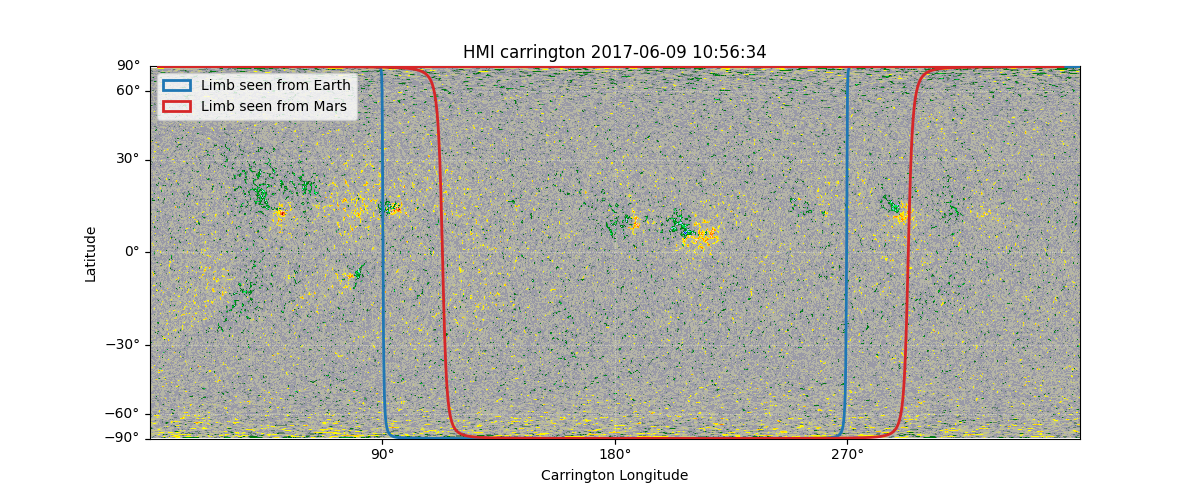# Plotting the solar limb¶

This example demonstrates how you can draw the limb as seen by an arbitrary observer.

```import matplotlib.pyplot as plt

import sunpy.map
from sunpy.coordinates import get_body_heliographic_stonyhurst
from sunpy.visualization import drawing
```

```syn_map = sunpy.map.Map('http://jsoc.stanford.edu/data/hmi/synoptic/hmi.Synoptic_Mr.2191.fits')
syn_map.plot_settings['cmap'] = 'hmimag'
syn_map.plot_settings['norm'] = plt.Normalize(-1500, 1500)
```

Get coordinates for Earth and Mars at the date of the synoptic map

```coords = {body: get_body_heliographic_stonyhurst(body, syn_map.date)
for body in ['Earth', 'Mars']}
```

Now we can plot the map the the solar limb seen from these two coordinates. To create a legend for these limbs, we need to keep the patches returned by `limb()` and provide them to `legend()`.

```fig = plt.figure(figsize=(12, 5))
im = syn_map.plot(axes=ax)

visible_limbs = []
for (body, coord), color in zip(coords.items(), ['tab:blue', 'tab:red']):
v, _ = drawing.limb(ax, coord, color=color, label=f'Limb seen from {body}', linewidth=2)
visible_limbs.append(v)

ax.legend(handles=visible_limbs)
plt.show()
``````INFO: Missing metadata for solar radius: assuming the standard radius of the photosphere. [sunpy.map.mapbase]
```

Total running time of the script: ( 0 minutes 3.331 seconds)

Gallery generated by Sphinx-Gallery